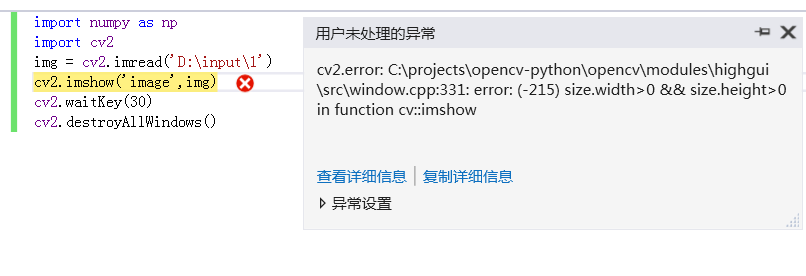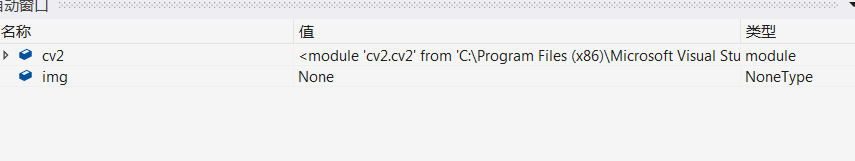python-opencv中imshow总报错的问题 5C

cv2.error
Message=C:\projects\opencv-python\opencv\modules\highgui\src\window.cpp:331: error: (-215) size.width>0 && size.height>0 in function cv::imshow

StackTrace:0

4个回答

0

0

1这个文件存在吗？是文件夹还是文件，这里应该是一个图片文件

0

0python-opencv里面图片无法imshow的处理
def thresholding(img): x_thresh = utils.abs_sobel_thresh(img, orient='x', thresh_min=180 ,thresh_max=255) mag_thresh = utils.mag_thresh(img, sobel_kernel=3, mag_thresh=(50, 100)) dir_...
OpenCV中imshow()出错问题

opencv使用imshow出错
opencv使用imshow出错
python学习笔记（七）多线程

python-opencv安装文件
python-opencv安装文件python-opencv安装文件python-opencv安装文件python-opencv安装文件

from sklearn impor tdatasets# 导入数据集模块import matplotlib.pyplot as plt# 导入绘图模块# 载入数据集digits_data = datasets.load_digits()# 绘制数据集前 5 个手写数字的灰度图for index, image in enumerate(digits_dat...
linux下cv2.imshow(）无法使用的解决方法
img = cv2.imread(image_path) plt.imshow(img, cmap = 'gray', interpolation = 'bicubic') plt.xticks([]), plt.yticks([]) # to hide tick values on X and Y axis plt.show() 转载：OpenCV error: the function ...
opencv 多线程显示linux 问题
opencv 多线程显示imshow出现图片不更新的问题1.在linux 下使用opencv多线程使用opencv的imshow显示图片时，很多时候会出现图片不更新的情况（但是实际内存数据在变化），经过多次试验后，发现一个可以暂时解决图片显示不更新方法：1 .首先将所有的图片显示放到一个线程中，在该线程中统一显示。2. 显示代码：Mat a;//a会不断的循环更新namedWindow("img"
opencv多线程读取视频的问题

tensorflow 一些奇怪的问题
import matplotlib.pyplot as plt filename = '1.jpg' with tf.gfile.FastGFile(filename,'rb') as f: image_buffer = f.read() image = tf.image.decode_jpeg(image_buffer) with tf.Session() as sess: im...
MATLAB中imshow()显示问题
MATLAB中imread(img),读取保存的数据是unit8类型的，如果需要运算，最好转换成double型，直接im2double(img)就可以，然后这样处理的数据全是[0,1]之间的。当然imshow(img),img不管是double型还是unit8 型都可以显示。imshow(I,[]); ----------- 自动调整数据的范围以便于显示 imshow(I,[low hi
VS中调用imshow报内存出错解决办法

matlab中imshow()出错问题

imshow与显示图像时的全白问题

ubuntu 16.04 OpenCV 2.4.13：在不同的线程中调用imshow失败
imshow是一个显示gui，在被一个线程调用时很可能也会被其他线程调用，存在冲突。 因此，在显示图像之前，线程必须锁定imshow，并在waitKey函数指定的一段时间。       如下        mtx.lock();        cv::imshow("img_left",img_left);         if((char)waitKey(5)=='s')
imshow()函数：参数是不同类型的情况

imshow()异常的解决办法

MALTAB中 imshow函数出错
imshow函数无法使用 / 错误使用 Settings / 未定义函数或变量 ‘matlab.internal.getSettingsRoot’。 具体问题\color{Blue}{\text{具体问题}}：imshow无法使用, 一使用,则报出以下提醒: 详细信息：\color{Blue}{\text{详细信息：}} 错误使用 Settings 未定义函数或变量 ‘matlab.int
OpenCV中 imshow函数运行中断的解决方法

python-opencv的安装方法及有可能的错误汇总（raspi）

OpenCV2.4.11调用摄像头显示拍摄视频出错

python中plt.imshow（img）显示不了图片解决方案

Matlab学习笔记（1）——imshow函数的使用
Matlab的imshow函数是用来显示图像的，但是其有几种不同的使用方式，使用不同的方式，会使显示的效果差别很大，在此对它的使用方式做一下说明
matlab中的imshow函数使用

Operation not permitted: ‘/var/folders执行：sudo pip install --user opencv-python

OpenCV中imshow函数的使用

opencv中图像连续显示的内存泄露问题的解决办法

OpenCV中imshow（）的几点说明

python中plt.imshow（img）显示不了图片
import openslide import numpy import pylab import matplotlib.pyplot as plt slide = openslide.OpenSlide('/media/xhj/LENOVO/data/Metastasis/Tumor_005.tif') tile = numpy.array(slide.read_region((0,0),6,...

1、imshow(f)和imshow(f,[ ])显示效果不同 imshow(f)直接显示f图像；imshow(f,[])显示的是灰度级数归一化后的图像，其中，f的最大值和最小值作为白(255)与黑(0)。 2、傅里叶变换的过程中是否要进行填充，根据冈萨雷兹的《数字图像处理》，在频率域滤波需要先对原始图像f(x,y)填0，然后再进行傅里叶变换。     经过实验验证发现，填充的图...
Matlab中显示图像imshow（A）与imshow（A,[]）的区别
imshow（A）与imshow（A,[]）两者都是显示图片，如果A的灰度都在20以下,但确实又有灰度变化,你直接imshow看起来几乎都是黑的,imshow(A,[])后会从最小到最大拉伸显示,使显示的图片对比度差距更明显，...

OpenCV问题集锦，图片显示不出来，WaitKey(0),imread()不能读图片，未经处理的异常，等问题集合

python-opencv安装过程，错误汇总

Mac 下安装 Python-OpenCV Python-OpenCV 处理图像（一）：基本操作